# GenEst - 2. Tutorial with Solar Example

### Introduction

In this vignette we walk through an example illustrating how GenEst command line utilities could be used to estimate mortality for different size birds at a large field of solar photovoltaic collectors. Our objective is to estimate overall mortality, as well as how mortality varies over time, whether it constant throughout the facility, and finally how different size classes of birds are affected.

The general steps in the analysis are:

1. Construct a model for Searcher Efficiency
2. Construct a model for Carcass Persistance
3. Estimate mortality
4. Specify the type of summary desired (for example, by season and species)

There are five files in total which make up the example dataset. For convenience, these files can be accessed in R as a list:

library(GenEst)
data(solar_PV)
names(solar_PV)
#>  "SE"  "CP"  "SS"  "DWP" "CO"

Alternatively, the files may be downloaded as .csv files from https://code.usgs.gov/ecosystems/GenEst/-/releases in the “For more info” section.

### Part 1: Searcher Efficiency Modeling

Searcher efficiency (SE) is modeled as a function of the number of times a carcass has been missed in previous searches and any number of covariates. The probability of finding a carcass that is present at the time of search is p on the first search after carcass arrival and is assumed to decrease by a factor of k each time the carcass is missed in searches. (For further background on field trials, and information about how to format the results for use with GenEst, see the User Guide, which is available at the code.usgs.gov page cited above).

Results of the SE field trials used in this example are stored in the data_SE data frame:

data_SE <- solar_PV$SE head(data_SE) #> seID Season Size Search1 Search2 Search3 Search4 Distance #> 1 se1 winter small 1 NA NA NA 17 #> 2 se2 winter small 1 NA NA NA 26 #> 3 se3 winter small 0 1 NA NA 54 #> 4 se4 winter small 0 1 NA NA 28 #> 5 se5 winter small 1 NA NA NA 46 #> 6 se6 winter small 1 NA NA NA 40 GenEst provides tools to construct and compare specific individual models, to explore which subsets of variables are most useful, and to automatically construct entire sets of models. To start we will fit a basic model in which the probability of detecting a carcass, p, and compounding difficulty to detect, k, depend only on their respective intercepts (and not other factors such as season or size). The function pkm is used to create a searcher efficiency model, which is returned as a pkm object. SE_model <- pkm(p ~ 1, k ~ 1, data = data_SE) SE_model #>$call
#> pkm0(formula_p = formula_p, formula_k = formula_k, data = data,
#>     obsCol = obsCol, kFixed = kFixed, kInit = kInit, CL = CL,
#>     quiet = quiet)
#>
#> $formula_p #> p ~ 1 #> #>$formula_k
#> k ~ 1
#>
#> $predictors #> character(0) #> #>$AICc
#>  489
#>
#> $convergence #>  0 #> #>$cell_pk
#>   cell   n p_median    p_lwr    p_upr k_median    k_lwr    k_upr
#> 1  all 240 0.664805 0.615057 0.711143 0.846097 0.742135 0.913056
#>
#> $CL #>  0.9 To explore whether use of covariate is warranted, pkm is used with the allCombos = TRUE. The specified model will be fit as will models formed using all combinations of predictors listed for the p and k parameters. orginal model. For example, p ~ Season can be simplified into p ~ 1, our original model in which p is independent of season. SE_model_set <- pkm(p~Season, k~1, data = data_SE, allCombos = TRUE) class(SE_model_set) #>  "pkmSet" "list" length(SE_model_set) #>  2 names(SE_model_set) #>  "p ~ Season; k ~ 1" "p ~ 1; k ~ 1" class(SE_model_set[]) #>  "pkm" "list" The set of models is contained in a pkmSet object. We could inspect the two models stored in the pkmSet individually, or for convenience we can view the AICc values simultaneously for all models using the function. Summary plots can be obtained by plotting any of the individual objects or the set as well. aicc(SE_model_set) #> p Formula k Formula AICc ΔAICc #> 2 p ~ 1 k ~ 1 489.00 0.00 #> 1 p ~ Season k ~ 1 492.11 3.11 Rather than one searcher efficiency model for all birds, it is often preferable to fit a seperate model for each size class. The sizeCol argument of the pkm function is the name of the column in data_SE that gives the size class for each carcass in the SE trials. If a sizeCol is provided, pkm returns a list of separate pk models fit for each size class. SE_size_model <- pkm(p ~ Season, k ~ 1, sizeCol = "Size", data = data_SE) class(SE_size_model) #>  "pkmSize" "list" names(SE_size_model) # A list is created with a model set per size class. #>  "lrg" "med" "small" class(SE_size_model$small)
#>  "pkm"  "list"
names(SE_size_model$small) # Each model set contains one model in this case. #>  "call" "data" "data0" "formula_p" "formula_k" #>  "predictors" "predictors_p" "predictors_k" "AIC" "AICc" #>  "convergence" "varbeta" "cellMM_p" "cellMM_k" "nbeta_p" #>  "nbeta_k" "betahat_p" "betahat_k" "levels_p" "cells" #>  "ncell" "cell_pk" "CL" "observations" "carcCells" #>  "loglik" "pOnly" "data_adj" To fit all combinations of models for each size class, use pkm with a sizeCol parameter and with allCombos = T. Once we have decided on which models to use for each size class, we store the corresponding pkm objects in a list for future use. In this case, we will choose the models with the lower AICc. SE_size_model_set <- pkm(p ~ Season, k ~ 1, sizeCol = "Size", data = data_SE, allCombos = TRUE) aicc(SE_size_model_set) #>$lrg
#>   p Formula  k Formula  AICc ΔAICc
#> 1 p ~ Season k ~ 1     95.65  0.00
#> 2 p ~ 1      k ~ 1     96.47  0.82
#>
#> $med #> p Formula k Formula AICc ΔAICc #> 2 p ~ 1 k ~ 1 178.62 0.00 #> 1 p ~ Season k ~ 1 182.40 3.78 #> #>$small
#>   p Formula  k Formula   AICc ΔAICc
#> 2 p ~ 1      k ~ 1     202.17  0.00
#> 1 p ~ Season k ~ 1     206.48  4.31
SE_models <- list()

Size small:

SE_models$small <- SE_size_model_set$small[]

Size Medium:

SE_models$med <- SE_size_model_set$med[]

Size Large:

SE_models$lrg <- SE_size_model_set$lrg[]

### Part 2: Carcass Persistence Modeling

A carcass persistence model estimates the amount of time a carcass would persist for, given the conditions under which it arrived. A number of carcasses have been placed in the field and periodically checked for scavanging. Results of the CP field trials used in this example are stored in the data_CP data frame:

data_CP <- solar_PV$CP head(data_CP) #> cpID Season Size LastPresent FirstAbsent #> 1 cp1 winter small 0.00 1.05 #> 2 cp2 winter small 21.02 27.97 #> 3 cp3 winter small 4.03 7.01 #> 4 cp4 winter small 0.00 0.97 #> 5 cp5 winter small 27.99 NA #> 6 cp6 winter small 10.02 13.99 and represent the left (start) and right (end) endpoints of the interval over which a carcass went missing. For further information about CP trials and how to format results for use with GenEst, see the User Guide (link found on help menu of the GUI, which can be accessed by entering runGenEst() from the R console). Four classes of parameteric models may be used for carcass persistance: exponential, Weibull, logistic, and lognormal. As with Searcher Efficiency we can fit one specific model, test a set of covariates and choose our favorite single model, or fit seperate models dependent on size class. First we will fit a single Weibull models for all birds. Weibull distributions have two parameters, location and scale. We will specify that the location depends on season by setting l ~ season, but scale only depends on the intercept using s ~ 1. cpm(l ~ Season, s ~ 1, data = data_CP, left = "LastPresent", right = "FirstAbsent", dist = "weibull") #>$call
#> cpm0(formula_l = formula_l, formula_s = formula_s, data = data,
#>     left = left, right = right, dist = dist, CL = CL, quiet = quiet)
#>
#> $formula_l #> l ~ Season #> #>$formula_s
#> s ~ 1
#>
#> $distribution #>  "weibull" #> #>$predictors
#>  "Season"
#>
#> $AICc #>  1051.61 #> #>$convergence
#>  0
#>
#> $cell_ls #> cell n l_median l_lwr l_upr s_median s_lwr s_upr #> 1 fall 60 2.473 2.087 2.859 1.637 1.457 1.838 #> 2 spring 60 2.801 2.395 3.208 1.637 1.457 1.838 #> 3 summer 60 2.689 2.296 3.083 1.637 1.457 1.838 #> 4 winter 60 2.509 2.127 2.891 1.637 1.457 1.838 #> #>$cell_ab
#>     cell  n pda_median pda_lwr pda_upr pdb_median pdb_lwr pdb_upr
#> 1   fall 60      0.611   0.544   0.686     11.858   8.061  17.444
#> 2 spring 60      0.611   0.544   0.686     16.461  10.968  24.730
#> 3 summer 60      0.611   0.544   0.686     14.717   9.934  21.824
#> 4 winter 60      0.611   0.544   0.686     12.293   8.390  18.011
#>
#> $CL #>  0.9 #> #>$cell_desc
#>     cell medianCP        r1        r3        r7       r14       r28
#> 1   fall 6.508736 0.8733664 0.7695707 0.6489244 0.5240883 0.3856577
#> 2 spring 9.035276 0.8948948 0.8063180 0.7000989 0.5856841 0.4518313
#> 3 summer 8.078012 0.8879564 0.7943671 0.6832539 0.5651042 0.4292548
#> 4 winter 6.747503 0.8759150 0.7738696 0.6548159 0.5310385 0.3929133

Next, we try a CP model set considering whether the season covariate for location is necessary, by comparing the l ~ season, s ~ 1 to l ~ 1, s ~ 1.

  CP_weibull_set <- cpm(l ~ Season, s ~ 1, data = data_CP,
left = "LastPresent",
right = "FirstAbsent",
dist = "weibull", allCombos = TRUE)
class(CP_weibull_set)
#>  "cpmSet" "list"
aicc(CP_weibull_set)
#>   Distribution Location Formula Scale Formula    AICc ΔAICc
#> 2 weibull      l ~ 1            s ~ 1         1046.65  0.00
#> 1 weibull      l ~ Season       s ~ 1         1051.61  4.96

Finally we will construct sets of CP models for each size class, however this time we will also consider models based on both exponential and weibull distributions. To compare models for multiple distributions, set dist to a vector of the distribution names to be considered. With a sizeCol provided and allCombos = TRUE, cpm returns a list of cpmSet objects, one for each size class.

CP_size_model_set <- cpm(formula_l = l ~ Season,
formula_s = s ~ 1,
left = "LastPresent",
right = "FirstAbsent",
dist = c("exponential", "weibull"),
sizeCol = "Size",
data = data_CP, allCombos = TRUE)
class(CP_size_model_set)
#>  "cpmSetSize" "list"
names(CP_size_model_set)
#>  "lrg"   "med"   "small"
class(CP_size_model_set$small) #>  "cpmSet" "list" length(CP_size_model_set$small)
#>  4
names(CP_size_model_set$small) #>  "dist: exponential; l ~ Season; NULL" "dist: exponential; l ~ 1; NULL" #>  "dist: weibull; l ~ Season; s ~ 1" "dist: weibull; l ~ 1; s ~ 1" We now have the flexibility to select models from different families for different size classes. We will choose to use the models with lower AICc, which requires storing the corresponding cpm objects in a list for later use. aicc(CP_size_model_set) #>$lrg
#>   Distribution Location Formula Scale Formula   AICc ΔAICc
#> 2 exponential  l ~ 1            NULL          294.59  0.00
#> 4 weibull      l ~ 1            s ~ 1         295.60  1.01
#> 1 exponential  l ~ Season       NULL          299.61  5.02
#> 3 weibull      l ~ Season       s ~ 1         300.86  6.27
#>
#> $med #> Distribution Location Formula Scale Formula AICc ΔAICc #> 4 weibull l ~ 1 s ~ 1 352.44 0.00 #> 3 weibull l ~ Season s ~ 1 356.47 4.03 #> 2 exponential l ~ 1 NULL 358.25 5.81 #> 1 exponential l ~ Season NULL 361.45 9.01 #> #>$small
#>   Distribution Location Formula Scale Formula   AICc ΔAICc
#> 4 weibull      l ~ 1            s ~ 1         346.02  0.00
#> 3 weibull      l ~ Season       s ~ 1         348.88  2.86
#> 1 exponential  l ~ Season       NULL          380.56 34.54
#> 2 exponential  l ~ 1            NULL          382.04 36.02
CP_models <- list()

Size small:

CP_models$small <- CP_size_model_set$small[]

Size med:

CP_models$med <- CP_size_model_set$med[]

Size lrg:

CP_models$lrg <- CP_size_model_set$lrg[]

### Part 3: Mortality Estimation

Estimating mortality requires bringing together models, carcass observation data (CO), and information on how the data was gathered. In particular the search schedule (SS) and proportion of carcasses in searchable areas (the density weighted proportion, or DWP), are needed. We will breifly inspect the files. Further information on the formatting of the CO, SS, and DWP files can be found in the User Guide.

Carcass observations:

data_CO <- solar_PV$CO head(data_CO) #> carcID Unit Species Size Row Distance DateFound X Y #> 1 x1 Unit82 SA small 28 13 2018-01-26 524641 3857110 #> 2 x2 Unit147 SA small 30 23 2018-01-13 525440 3857592 #> 3 x3 Unit235 SA small 11 44 2018-03-18 526724 3858148 #> 4 x4 Unit213 SA small 31 44 2018-02-04 526408 3858069 #> 5 x5 Unit204 SA small 34 10 2018-04-29 524953 3858081 #> 6 x6 Unit117 SA small 25 27 2018-04-06 527023 3857256 Search schedule: data_SS <- solar_PV$SS
data_SS[1:5 , 1:10]
#>   DateSearched Season Unit1 Unit2 Unit3 Unit4 Unit5 Unit6 Unit7 Unit8
#> 1   2017-12-20 winter     1     1     1     1     1     1     1     1
#> 2   2017-12-21 winter     1     1     1     1     1     1     1     1
#> 3   2017-12-22 winter     0     0     0     0     0     0     0     0
#> 4   2017-12-23 winter     0     0     0     0     0     0     0     0
#> 5   2017-12-24 winter     0     0     0     0     0     0     0     0

(Note that there are 300 arrays columns altogether: Unit1, …, Unit300)

Density weighted proportion:

data_DWP <- solar_PV$DWP head(data_DWP) #> Unit small med lrg #> 1 Unit1 1 1 1 #> 2 Unit2 1 1 1 #> 3 Unit3 1 1 1 #> 4 Unit4 1 1 1 #> 5 Unit5 1 1 1 #> 6 Unit6 1 1 1 These elements combine in the function estM, producing an object containing simulated arrival, detection, and mortality distributions. We also have the opportunity to provide the fraction of the facility being surveyed, frac, if it happens to be less than 100%. Increasing the number of simulations, nsim, will improve the accuracy of the estimates but comes at a cost of computer runtime. When estimating mortality, it is not currently possible to mix CP and SE models which differ in their dependence on size. Either both models depend on size class, or both models must be independent of size class. In this case we will choose here to use size dependence.  Mest <- estM( nsim = 100, frac = 1, data_CO = data_CO, data_SS = data_SS, data_DWP = data_DWP, model_SE = SE_models, model_CP = CP_models, unitCol = "Unit", sizeCol = "Size", COdate = "DateFound", SSdate = "DateSearched" ) We are now able to get a confidence interval for estimated total mortality by taking summary of the estM object. Plotting it shows us us the estimated probability density for number of fatalities. plot(Mest)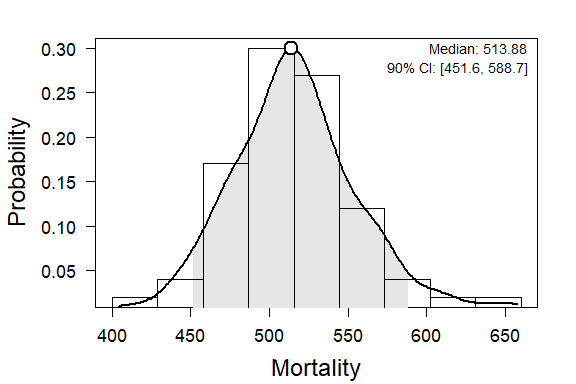A point estimate for overall sitewide mortality is listed at the top of the plot, satisfying our first objective. The period of inference only covers the period over which we have have fatality monitoring data, which in this case is from 2017-12-20 to 2018-12-19. ### Part 4: Summaries Having calculated the estimated arrival densities for each of the carcases, we can now use them to create a variety of summaries. Suppose that we are interested in how mortality changes with respect to three kinds of variables: 1. Temporally - by season, or finer resolutions 2. Spatially - summaries by search unit 3. Among Size classes, or other groups To create summaries, we split the data by differnt covariates, using a function called calcSplits. This requires the simulated mortality $Mhat and arrival times $Aj stored in the estM object, plus the search schedule and carcass observation data. Splits to the search schedule (splits in time) are specified by assigning a covariate to split_SS. These must be variables present in the Search Schedule file. To investigate differences in mortality between season, we will set split_SS to Season. unique(data_SS[, "Season"]) #>  "winter" "spring" "summer" "fall" M_season <- calcSplits(M = Mest, split_SS = "Season", data_SS = data_SS, split_CO = NULL, data_CO = data_CO ) Splitting the estM creates a splitFull object, a plot of which shows boxplots for each season. plot(M_season)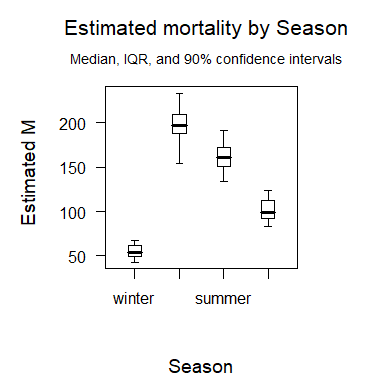Taking a summary of the splitFull object gives us a confidence interval for each level of the split covariate. The size of the confidence interval can be specified for both plots or summaries using the CL argument. summary(M_season, CL = 0.95) #> X 2.5% 25% 50% 75% 97.5% #> winter 28.93857 41.18001 49.33988 54.37020 61.80688 73.70444 #> spring 84.89571 147.72201 187.83375 196.91397 209.54065 246.83686 #> summer 73.70714 130.48043 151.19795 161.34303 172.40153 205.64579 #> fall 45.45857 82.43028 91.69147 98.51951 112.46304 125.49912 #> attr(,"CL") #>  0.95 #> attr(,"vars") #>  "Season" #> attr(,"type") #>  "SS" #> attr(,"times") #>  0 89 182 276 364 #> attr(,"class") #>  "splitSummary" To get a finer summary of mortality, we need to parse the search schedule, using the function prepSS. This allows us to specify the exact time intervals over which we will split, in this case we will create a weekly summary.  SSdat <- prepSS(data_SS) # Creates an object of type prepSS. schedule <- seq(from = 0, to = max(SSdat$days), by = 7)
tail(schedule)
#>  329 336 343 350 357 364

When we plot the splitFull object for a split with a custom schedule, we must specify that the rate is per split catagory by setting rate = T.

M_week <- calcSplits(M = Mest,
split_time = schedule,
data_SS = SSdat,
data_CO = data_CO
)
plot(x = M_week, rate = TRUE)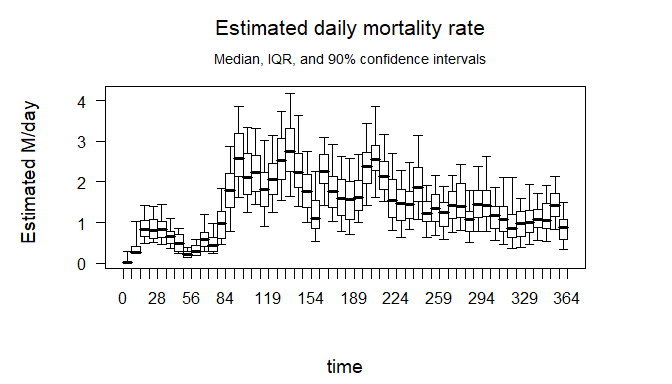Next we will look at at splitting by covariates present in the Carcass Observation file. We specify a CO split by assigning split_CO to the name (or names) of the variables we wish to split on. Suppose we would like a summary of estimated mortality by unit.


M_unit <- calcSplits(M = Mest,
split_CO = "Unit",
data_CO = data_CO,
data_SS = data_SS
)
plot(M_unit, rate = FALSE)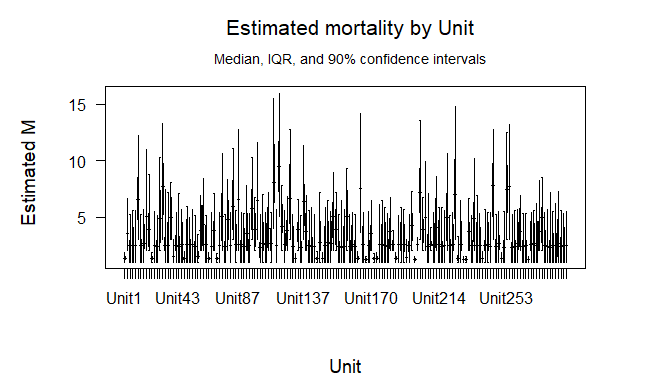There are 300 units in this example, each one gets a boxplot when we plot the splitFull. For those arrays which have at least one observation, we can create a summary. In this case we will only create a summary for arrays 8 and 100.

dim(summary(M_unit))  # only 164 arrays had observations.
#>  164   6

# A list of the arrays without observed carcasses:
setdiff(paste0("Unit", 1:300), data_CO\$Unit)
#>    "Unit2"   "Unit3"   "Unit4"   "Unit5"   "Unit9"   "Unit13"  "Unit17"
#>    "Unit19"  "Unit20"  "Unit24"  "Unit25"  "Unit28"  "Unit29"  "Unit34"
#>   "Unit35"  "Unit36"  "Unit37"  "Unit38"  "Unit39"  "Unit40"  "Unit41"
#>   "Unit42"  "Unit45"  "Unit47"  "Unit48"  "Unit51"  "Unit55"  "Unit56"
#>   "Unit57"  "Unit59"  "Unit60"  "Unit62"  "Unit63"  "Unit66"  "Unit69"
#>   "Unit70"  "Unit71"  "Unit73"  "Unit74"  "Unit75"  "Unit81"  "Unit84"
#>   "Unit85"  "Unit86"  "Unit88"  "Unit90"  "Unit91"  "Unit93"  "Unit94"
#>   "Unit95"  "Unit97"  "Unit99"  "Unit102" "Unit103" "Unit104" "Unit105"
#>   "Unit108" "Unit109" "Unit111" "Unit119" "Unit120" "Unit121" "Unit122"
#>   "Unit124" "Unit125" "Unit127" "Unit128" "Unit132" "Unit133" "Unit136"
#>   "Unit143" "Unit146" "Unit148" "Unit149" "Unit151" "Unit164" "Unit166"
#>   "Unit169" "Unit172" "Unit175" "Unit179" "Unit181" "Unit185" "Unit186"
#>   "Unit188" "Unit189" "Unit191" "Unit192" "Unit193" "Unit197" "Unit199"
#>   "Unit200" "Unit201" "Unit202" "Unit205" "Unit209" "Unit211" "Unit216"
#>   "Unit221" "Unit222" "Unit225" "Unit226" "Unit228" "Unit231" "Unit233"
#>  "Unit234" "Unit236" "Unit246" "Unit247" "Unit249" "Unit251" "Unit254"
#>  "Unit255" "Unit256" "Unit257" "Unit259" "Unit261" "Unit262" "Unit264"
#>  "Unit266" "Unit267" "Unit272" "Unit274" "Unit275" "Unit280" "Unit282"
#>  "Unit283" "Unit285" "Unit288" "Unit289" "Unit291" "Unit293" "Unit294"
#>  "Unit295" "Unit297" "Unit298"

# Create summaries for arrays Unit12 and Unit100.
whichRow <- rownames(summary(M_unit))  %in% c("Unit12", "Unit100")
summary(M_unit)[whichRow, ]
#>         X 5%      25%      50%      75%       95%
#> Unit12  1  1 1.000000 2.436138 2.966872  5.251306
#> Unit100 3  3 3.852121 5.774465 7.425038 10.325280

It is possible to create summaries that split on both Carcass Observation variables and Search Schedule variables. To do so, include both a split_SS and a split_CO argument.


M_unit_and_species <- calcSplits(M = Mest,
split_SS = c("Season"),
split_CO = c("Size"),
data_CO = data_CO,
data_SS = data_SS
)
plot(M_unit_and_species, rate = FALSE)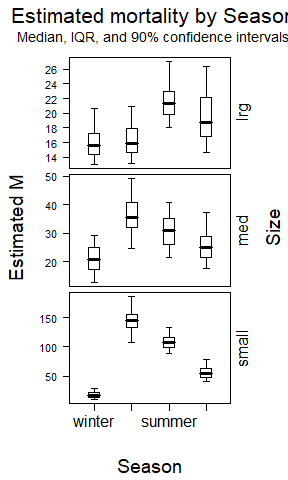Two CO variables can be compared simultaneously by specifying an ordered pair of covariates for split_CO, however currently there are a limited total number (two) of splits which can be allocated among temporal or carcass covariates.